Home MonkeyNotes Printable Notes Digital Library Study Guides Study Smart Parents Tips College Planning Test Prep Fun Zone Help / FAQ How to Cite New Title Request

 8.3 Slope and intercepts Between the graph of a linear equation and the equation itself, one can have two types of relationships I) The slope of the straight line II) Its intercepts. Slope: In mathematics the word 'slope' has a specific meaning (see figure). Thus slope of segment MN is the ratio of the difference between y - coordinates of point M and N to the corresponding difference between their x - coordinates respectively. Slope of segment MN =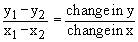Where x1 ą x2 means that the x - coordinates of both M and N should be different. But if x1 = x2 then the segment is parallel to y - axis i.e. it is a vertical segment which has no slope. Slope of any non-vertical segment can be always found. Slope of any line is the slope of any segment of it.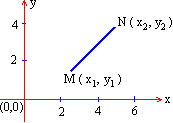Note that : Slopes of parallel lines are equal. The slopes of a line parallel to the x - axis and the x - axis itself is zero. (Horizontal lines). The slope of a line parallel to the y - axis and the y - axis itself has no slope or this slope is undefined. (vertical lines). A line having a positive slope makes an acute angle with the positive direction of the x - axis. A line having a negative slope, makes an obtuse angle with the positive direction of the x - axis. The products of slopes of two mutually perpendicular lines is always -1 i.e. if two mutually perpendicular lines have slopes m1 and m2 respectively then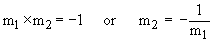Example Find slope 'm' of the straight line through (-3, 2) and (5, -2) Solution : (x1, y1) = (-3, 2) and (x2,y2) = (5,-2) Therefore slope (m) =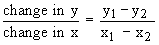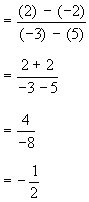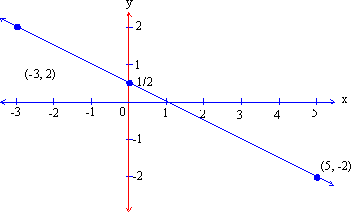Index 8.1 Definition8.2 Relations, Graphs and Symmetry8.3 Slopes and Intercepts Chapter 9
 Search: All Products Books Popular Music Classical Music Video DVD Toys & Games Electronics Software Tools & Hardware Outdoor Living Kitchen & Housewares Camera & Photo Cell Phones Keywords: